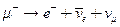Chapter 33, Problem 16PE

Chapter
Section
Textbook Problem

The decay mode of the negative muon is(a) Find the energy released in MeV.(b) Verify that charge and lepton family numbers are conserved.

To determine

(a)

The energy released in the decay of μ particle given by μe+ν¯e+νμ

Explanation

Given:

The decay mode of negative muon is given by, μe+ν¯e+νμ

Formula used:

Energy released = Rest energy of initial particle − sum of energies of decay products

Calculation:

In this problem the negative muon decays by μe+ν¯e+νμ

Rest energy of μ particle =105

To determine

(b)

The conservation of charge and lepton family numbers.

Still sussing out bartleby?

Check out a sample textbook solution.

See a sample solution

The Solution to Your Study Problems

Bartleby provides explanations to thousands of textbook problems written by our experts, many with advanced degrees!

Get Started

________ is lifes primary source of energy. a. Food b. Water c. Sunlight d. ATP

Biology: The Unity and Diversity of Life (MindTap Course List)

Why is degenerate matter so difficult to compress?

Horizons: Exploring the Universe (MindTap Course List)

Why do biologists often use model organisms in their research?

Biology: The Dynamic Science (MindTap Course List)

How far back in time can the memory of the ocean (sediments) provide information?

Oceanography: An Invitation To Marine Science, Loose-leaf Versin